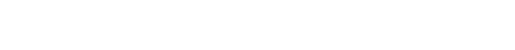### 02亲和性分析案例

```（1）defaultdict(int)：初始化为 0
（2）defaultdict(float)：初始化为 0.0
（3）defaultdict(str)：初始化为 ''```

### 03代码实现

```import  numpy as np
from collections import defaultdict
dataset_filename = "affinity_dataset.txt"
print(X[:5])   #打印前五行的购物信息``````num_apple_purchases = 0 # 初始化一个购买苹果人数的变量
for sample in X:
if sample == 1:
num_apple_purchases+=1
print("{0} people bought Apples ".format(num_apple_purchases))
num_banana_purchases = 0
for sample in X:
if sample == 1:
num_banana_purchases += 1
print("{0} people bought banana".format(num_banana_purchases))``````valid_rules = defaultdict(int)
invalid_rules = defaultdict(int)
num_occurances = defaultdict(int)
for sample in X:
for premise in range(4):
if sample[premise] ==0 :
continue
num_occurances[premise] +=1  #当顾客有购买物品时key对应的时value变为1
for conclusion in range(4):
if premise == conclusion:  #访问同一个key 的时候是没有意义的直接跳过
continue
if sample[conclusion] == 1:
valid_rules[(premise,conclusion)] +=1
else:
invalid_rules[(premise,conclusion)] +=1```

```support = valid_rules
#置信度的计算方法类似，遍历每条规则进行计算
confidence = defaultdict(float)
for premise,conclusion in valid_rules.keys():
rule = (premise,conclusion)
confidence[rule] = valid_rules[rule]/num_occurances[premise]```

```def print_rule(premise, conclusion,support , confidence,features):
premise_name = features[premise]
conclusion_name = features[conclusion]
print(" - Support : {0}".format(support[(premise,conclusion)]))
print(" - Confidence : {0:.3f}".format(confidence[(premise,conclusion)]))
premise = 1
conclusion = 3
print_rule(premise,conclusion,support,confidence,features)

from  operator import itemgetter
sorted_support = sorted(support.items(),key=itemgetter(1),reverse=True)```

```for index in range(5):
print("Rule #{0}".format(index+1))
premise,conclusion = sorted_support[index]
print_rule(premise,conclusion,support,confidence,features)```